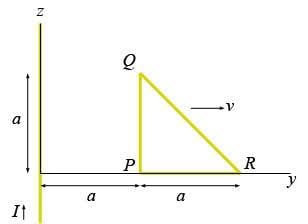# Missing minus sign - Motional EMF

Nick89

## Homework Statement

An infinitely long conductor carrying a current I in the positive z-direction lies on the z-axis.
A conducting triangle of wires lies in the yz-plane, which moves with a speed v in the positive y-direction.
The following figure shows the situation on time t = 0:a) Calculate the induced voltages $V_Q-V_P$ and $V_P-V_R$ on t=0.
I got this, no problem.

b) Calculate the induced voltage $V_Q-V_R$ on time t=0.
Hint: First calculate the induced voltage on a little segment dl and notice that $dl = \sqrt{dz^2 + dy^2} = \sqrt{2} dy$.

## Homework Equations

$$\epsilon = \int (\vec{v} \times \vec{B}) \cdot \vec{dl}$$
$$B = \frac{ \mu_0 I}{2 \pi y}$$ (at a distance y, in the negative x-direction (into the screen)

## The Attempt at a Solution

I got the answer right apart from a minus sign...

I first checked the direction of the crossproduct v*B and got it to be in the positive z-direction.
Dot product with dl is then
$$( \vec{v} \times \vec{B} ) \cdot \vec{dl} = vB \, dl \cos \theta = vB \, dl cos(135) = -\frac{1}{2} \sqrt{2} vB \, dl$$
Because dl is pointing from Q to R, the angle is 90 + 45 = 135, right?

Then:
$$\epsilon = -\frac{1}{2} \sqrt{2} \int_a^{2a} B v dl = -\frac{1}{2} \sqrt{2} \int_a^{2a} \frac{\mu_0 I}{2 \pi y} v \sqrt{2} dy = - \frac{\mu_0 I v}{2 \pi} \int_a^{2a} \frac{dy}{y} = - \frac{\mu_0 I v}{2 \pi} \ln{2}$$

As I said, the answer is correct apart from the minus sign... Where did I go wrong?

The answer I am given is this exactly:
$$d \epsilon = v \frac{\mu_0 I}{2 \pi y} \frac{1}{2}\sqrt{2} dl$$
$$V_Q-V_R = \int_a^{2a}\frac{\mu_0 I v}{2 \pi y} dy = \frac{ \mu_0 I v}{2 \pi } \ln{2}$$
That's all, nothing more... I can't quite figure out what they are doing, which equations they are using...

Last edited: